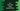# C++ program to find LCM using a separate function## C++ program to find LCM using a separate function:

In this post, we will learn how to find the LCM of two numbers in C++ by using a separate function. LCM is also called least common multiple, is the lowest common multiples of two numbers.

It is the smallest number which is divisible by both numbers.

Let’s take a look at the programs.

## Find LCM in C++:

``````#include <iostream>
using namespace std;

int findLCM(int firstNumber, int secondNumber)
{
int firstMultiple = firstNumber;
int secondMultiple = secondNumber;

while (firstMultiple != secondMultiple)
{
if (firstMultiple < secondMultiple)
{
firstMultiple += firstNumber;
}
else
{
secondMultiple += secondNumber;
}
}

return firstMultiple;
}

int main()
{
int first, second;

cout << "Enter the first number : " << endl;
cin >> first;

cout << "Enter the second number : " << endl;
cin >> second;

int lcm = findLCM(first, second);

cout << "LCM: " << lcm << endl;
}``````

Here,

• We are reading the numbers as inputs from the user and storing these in first and second variables.
• findLCM function is used to find the lcm of two numbers. It takes two integers as arguments and returns the LCM. This value is stored in the variable lcm.
• Inside findLCM, we have created two variables firstMultiple and secondMultiple to keep the multiples of the first and the second number.
• The while loop runs until the multiples of both numbers become equal. Inside the loop, we are incrementing the multiple, whichever is the smaller.
• Once the loop will end, firstMultiple and secondMultiple both will hold equal values. We can return any one of these value.

If you run this program, it will give output as like below:

``````Enter the first number :
11
Enter the second number :
12
LCM: 132

Enter the first number :
9
Enter the second number :
99
LCM: 99``````